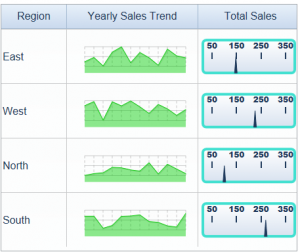The C1GridView template column allows you to host various controls inside the grid. Imagine a sales report with a grid where textual data is not that explanatory compared to visual representation. It would be so nice to see a chart showing sales trend inside a grid or gauge depicting total sales. You can achieve this simply by using template column of gridview and hosting controls within. Let me walk you through a simple application showing how to create one like below.For data we will need a sales and sales data class. The code for them is as below

``````public class Sales
{
public string Region { get; set; }
public string Month { get; set; }
public double Amount { get; set; }
}
public class SalesData : List<Sales>
{
public SalesData()
{
var cl = new CultureInfo("en-US");
List<string> monthsList = new List<string>();
Random rn = new Random(1000);
rn.Next(1000, 3000);
foreach (string month in monthsList)
{
this.Add(new Sales { Region = "East", Month = month, Amount = rn.Next(1000, 3000) });
this.Add(new Sales { Region = "West", Month = month, Amount = rn.Next(1500, 2500) });
this.Add(new Sales { Region = "North", Month = month, Amount = rn.Next(1000, 2500) });
this.Add(new Sales { Region = "South", Month = month, Amount = rn.Next(2000, 3000) });
}

}
}
``````
1. Place a C1GridView control on the page and click on the smart-tag and go to property builder.
2. Add one bound field and two template fields. Name them "Region", "Yearly Sales Trend" and "Total Sales" respectively.
3. In the "Yearly Sales Trend" template field and a item template and drop a C1LineChart
4. In the "Total Sales" template field add a item template and drop a C1LinearGauge.
5. Add event "OnRowDataBound" to the C1GridView.

The mark-up would like this.

``````<wijmo:C1GridView ID="C1GridView1" runat="server" AutogenerateColumns="false" OnRowDataBound="C1GridView1_RowDataBound" width="450" Height="375">
<Columns>
<wijmo:C1BoundField DataField="Region" HeaderText="Region" Width="100">
</wijmo:C1BoundField>
<wijmo:C1TemplateField HeaderText="Yearly Sales Trend" Width="200">
<ItemTemplate>
<wijmo:C1LineChart ID="saleschart" Width="250" Height="80" runat="server" AutoResize="true" Type="Area"></wijmo:C1LineChart>
</ItemTemplate>
</wijmo:C1TemplateField>
<wijmo:C1TemplateField HeaderText="Total Sales" Width="160">
<ItemTemplate>
<wijmo:C1LinearGauge ID="salesGauge" runat="server" Min="50" Max="350" TickMajor-Interval="100" Width="150" Height="60" Face-FaceStyle-Stroke="Turquoise" Face-FaceStyle-Fill-Type="Default" Orientation="Horizontal">
</wijmo:C1LinearGauge>
</ItemTemplate>
</wijmo:C1TemplateField>
</Columns>
</wijmo:C1GridView>
``````

6. Define an instance of SalesData and Initialize the Sales data in page load event. 7. We will bind the grid to data grouped by "Region", so lets a create a linq query for that .

``````data = new SalesData();
var cdata=from c in data
group c by c.Region into cgp
select new
{
Region=cgp.First().Region,
Amount=cgp.Sum(x=>x.Amount),
Month=cgp.First().Month
};
``````

8. Assign C1GridView datasource to it.

``````this.C1GridView1.DataSource = cdata.ToList();

this.C1GridView1.DataBind();
``````

9. Next we will plot the line chart with yearly sales data in the "RowDataBound" event. We will again create a linq query for that. Each row will show sales trend for that specific region. We will also create chart data bindings here and map the fields. Here is the code listing.

``````if (e.Row.RowType == C1.Web.Wijmo.Controls.C1GridView.C1GridViewRowType.DataRow)
{
string region = DataBinder.Eval(e.Row.DataItem, "Region").ToString();
C1LineChart chart = (C1LineChart)e.Row.FindControl("saleschart");
var chartdata = from cdata in data
where cdata.Region == region
select cdata;
//Create Chart bindings
C1ChartBinding chartbinding = new C1ChartBinding();
chartbinding.YField = "Amount";
chartbinding.XField = "Month";
chartbinding.LegendEntry = false;
chart.DataSource = chartdata;
chart.DataBind();
chart.Axis.X.GridMajor.Visible = true;
chart.Axis.Y.GridMajor.Visible = true;
chart.Axis.Y.Visible = false;
chart.Axis.X.Visible = false;
chart.Axis.X.TextVisible = false;
chart.Axis.Y.TextVisible = false;
chart.ShowChartLabels = false;
``````

10. We also need to show Total Sales for that specific region. For this the linear gauge will be used and the value set according to region. Note that for simplicity the value is scaled down.

``````C1LinearGauge gauge = (C1LinearGauge)e.Row.FindControl("salesGauge");
gauge.Value=data.Where(x=>x.Region==region).Select(x=>x.Amount).First()/10;

}
``````

11. Now run the sample and you should see the chart and linear gauge bound to the C1GridView template column. Thank you for reading.